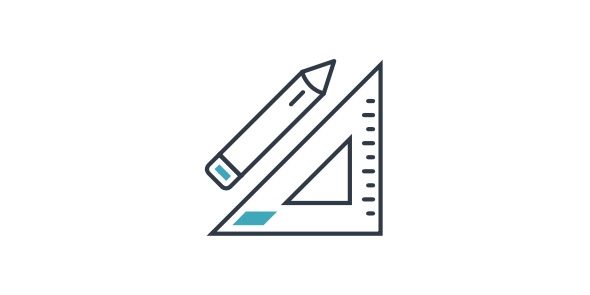15 Questions | Total Attempts: 231SettingsTake this ultimate quiz on 3rd grade math. It's pretty difficult so you might need extra time to score a perfect.

• 1.
What is 4648 rounded to the nearest 10?
• A.

4650

• B.

4640

• C.

4670

• D.

4630

• 2.
What is 1 x 9?
• A.

0

• B.

1

• C.

9

• D.

9-Jan

• 3.
What is 500 + 7?
• A.

577

• B.

507

• C.

493

• D.

570

• 4.
What is an estimate for 53 + 36?
• A.

60

• B.

80

• C.

70

• D.

90

• 5.
What is 7 + 30 + 600?
• A.

367

• B.

736

• C.

637

• D.

376

• 6.
What pair of numbers have a sum of 12 and a product of 35?
• A.

4, 8

• B.

6, 6

• C.

5, 7

• D.

3, 9

• 7.
What is the largest number you can make from the digits 3, 9, 2, and 7?
• A.

9732

• B.

9723

• C.

9327

• D.

9237

• 8.
How do you write "four thousand four hundred forty-four" in standard form?
• A.

4044

• B.

4444

• C.

4404

• D.

444

• 9.
What is 4648 rounded to the nearest 100?
• A.

4700

• B.

4500

• C.

4800

• D.

4600

• 10.
What number is greater than 5 and less than 7?
• A.

6

• B.

8

• C.

9

• D.

7

• 11.
What is 47 x 4?
• A.

158

• B.

188

• C.

178

• D.

168

• 12.
What is 5456 - 4372?
• A.

1184

• B.

1094

• C.

1084

• D.

984

• 13.
What is the remainder when we divide 30 by 4?
• A.

4

• B.

5

• C.

3

• D.

2

• 14.
If a horse eats 7 pounds of food in 1 day, how much does it eat in 5 days?
• A.

49 Pounds

• B.

28 Pounds

• C.

35 Pounds

• D.

42 Pounds

• 15.
What is 600 + 50 + 3?
• A.

653

• B.

650

• C.

603

• D.

663

Related Topics